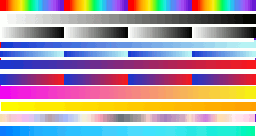# Colour coding a range of values of f( x, y)NB This program calls dlls, so is LB only, although you can use RB if you are prepared to load the bmp as a binary file and look up each pixel...

To demonstrate ways of colour coding smoothly a function of x and y, I calculate it (scaled to range f( x, y) >=0 and <=72 in this case), then draw it with different colour ranges. Some uniquely represent each height by one colour; others here cycle four times, so any one colour could represent any one of four heights. You gain in dramatic effect, but lose on interpretation! The red dot shows which colour gradient it is currently using.

The surface involved is simply equal to the radius, so represents a conical depression.

The colour scales were created with graduated fills in the GIMP (any paint software would do) and saved as a 24 bit bmp, which LB can load and interrogate pixel by pixel to get the rgb values. This is much more flexible than any method relying on calculating r g and b from f( x, y).

Which do YOU think best represents the surface? Five are uni-valued. The others are multi-valued. The program automatically saves them as BMPs.```    nomainwin

UpperLeftX    = 200
UpperLeftY    = 100
WindowWidth   = 450
WindowHeight  = 200

graphicbox #w.g0,  10, 10, 256, 140
graphicbox #w.g1, 320, 30, 100, 100
graphicbox #w.g2, 280, 10,  20, 140

dim ColLookUp\$( 256, 12), v( 12)

open "3D surface" for graphics_nsb as #w

#w.g1, "down ; fill black"

#w, "trapclose [quit]"

handleg0 =hwnd( #w.g0)
calldll #user32, "GetDC", uhandleg0 as long, hDC as ulong

#w.g0, "down ; drawbmp Scale 0 0"
#w.g0, "flush"

for l =1 to 11                                  '   since my bmp has 11 graduated colour stripes
read yy                                 '   which is how far down next colour stripe is centred
v( l) =yy
for v =0 to 255
calldll #gdi32, "GetPixel",_
hDC as ulong,_
v   as long,_
yy  as long,_
pixcol as long

bl = int(  pixcol /( 256*256))
gr = int( (pixcol               -bl *256*256) / 256)
re = int(  pixcol               -bl *256*256 - gr *256)

ColLookUp\$( v, l) =str\$( re) +" " +str\$( gr) +" " +str\$( bl)
next v
scan
next l

callDll #user32, "ReleaseDC",_
handleg0 as ulong,_                          '   handle of graphic box
hDC as ulong,_                               '   dc from GetDC
result as ushort                            '   returns non-zero if successful

c =-1

[start]
timer 0
c =( c +1) mod 11                               '   choose in turn each of 11 colour sequences. ( c =0 to 10)
ch =c +1                                        '       ch =1 to 11
#w.g2 "goto 10 "; v( ch); " ; down ; color red ; backcolor red ; circlefilled 5 ; up"
for x =0 to 99
scan
for y =0 to 99
r =( ( x -50)^2 +( y -50)^2)^0.5        '   r is radius from centre, between 0 and about 71
r =int( r)

#w.g1 "color "; ColLookUp\$( int( r /72 *255), ch)

#w.g1 "set ";  x; " "; y
next y
next x

sv\$ ="Bump"; str\$( ch)
#w.g1 "flush ; getbmp "; sv\$; " 0 0 99 99"
bmpsave sv\$, sv\$+".bmp"

#w.g2 "goto 10 "; v( ch); " ; down ; color white ; backcolor white ; circlefilled 4 ; up"
timer 2000, [start]
wait

data 5, 18, 33, 44, 53, 62, 80, 93, 105, 118, 129

[quit]